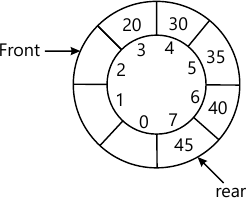MORE IN Data Structures and Applications
VTU Computer Science (Semester 3)
Data Structures and Applications
December 2011
Total marks: --
Total time: --
INSTRUCTIONS
(1) Assume appropriate data and state your reasons
(2) Marks are given to the right of every question
(3) Draw neat diagrams wherever necessary

1 (a) What is an algorithm? Briefly explain the criteria that an algorithm must satisfy.
8 M
1 (b) Write a recursive function to implement binary search.
8 M
1 (c) Define 'Big Oh' notation. Show that 3n+2=O(n) is correct.
4 M

2 (a) Develop a structure to represent planets in the solar system. Each planet has fields for the planets name, its distance from the sum in miles and the number of moons it has. Write a program to read the data for each planet and store. Also print the name of the planet that has the highest number of moons.
8 M
2 (b) For the given sparse matrix and its transpose, give that the triplet representation using one dimensional array. a is the given sparse matrix, b will be its transpose.
[ a=egin{bmatrix}15 &0 &0 &22 &0 &-15 \0 &11 &3 &0 &0 &0 \0 &0 &0 &-6 &0 &0 \0 &0 &0 &0 &0 &0 \91 &0 &0 &0 &0 &0 \0 &0 &28 &0 &0 &0 end{bmatrix} ]
8 M
2 (c) Consider two polynomials A(x) =2x1000+1 and B(x)=x4+10x3+3x2+1, show diagrammatically how these polynomial can be stored in a single 1-D array. Also give its C representation..
4 M

3 (a) Define stack. Give the C implementation of push and pop functions. Include check for empty and full condictions of stack.
8 M
3 (b) Write a C function to evaluate a postfix expression.
8 M
3 (c) For the given circular queue shown in fig.Q2(c), write the values of front and rear in the table after each specified operation is performed. Queue full/empty conditions must be considered. 0-7 indicate the array indices.

 Operation Rear Front Insert 0 Insert 10 Insert 15 delete4 M

4 (a) Explain how a chain can be used to implement a queue. Write the functions to insert and delete elements from such a queue.
8 M
4 (b) Describe the doubly linked lists with advantages and disadvantages. Write a C function to delete a node from a doubly linked list. Ptr is the pointer which points to the node to be deleted. Assume that there are nodes on either side of the node to be deleted.
8 M
4 (c) For the given sparse matrix, give the diagrammatic linked representation. [ a=egin{bmatrix} 0&1 &2 \3 &0 &0 \0 &0 &0 end{bmatrix} ]
4 M

5 (a) With reference to the Fig.Q5(a), answer the following:
i) Is it a binary tree?
ii) Is it a complete tree?
iii) Give the preorder traversal.
iv) Give the inorder traversal.
v) Give the postorder traversal.
vi) Give the list notation (using pairs of round brackets).
vii) Where will be the left child of node 4 pointing to, if it is converted to a threaded b-tree.
viii) Is it a max heap?
8 M
5 (b) Write the following C functions for
i) Counting the number of leaf nodes in a b-tree.
ii) Finding the inorder successor of a node in a threaded b-tree.
8 M
5 (c) Show that for any non-empty b-tree T, if n0 is the number of leaf nodes and n2 is the number of nodes of degree 2, then n0-n2+1.
4 M

6 (a) Explain the following with an example:
i) Forest ii) Graph iii) Winner tree
8 M
6 (b) Describe the binary search tree with an example. Write a iterative function to search for a key value in a binary search tree.
8 M
6 (c) Construct the b-tree from the given traversals:
Preorder : ABDCEF
Inorder : BDAEFC
Postorder : DBFECA
4 M

7 (a) Briefly explain the following with examples:
i) HBLT ii) WBLT
8 M
7 (b) What is binomial heap? Explain the step involved in the deletion of min element fromm= a binomial heap.
8 M
7 (c) Define Fibonacci heap. Briefly explain the different types.
4 M

8 (a) Describe the follwing with an example each:
i) height balanced trees ii) Optimal bst
8 M
8 (b) Explain the Red-black tree. State its properties.
8 M
8 (c) What is a splay tree? Briefly explain the different types of splay trees.
4 M

More question papers from Data Structures and Applications